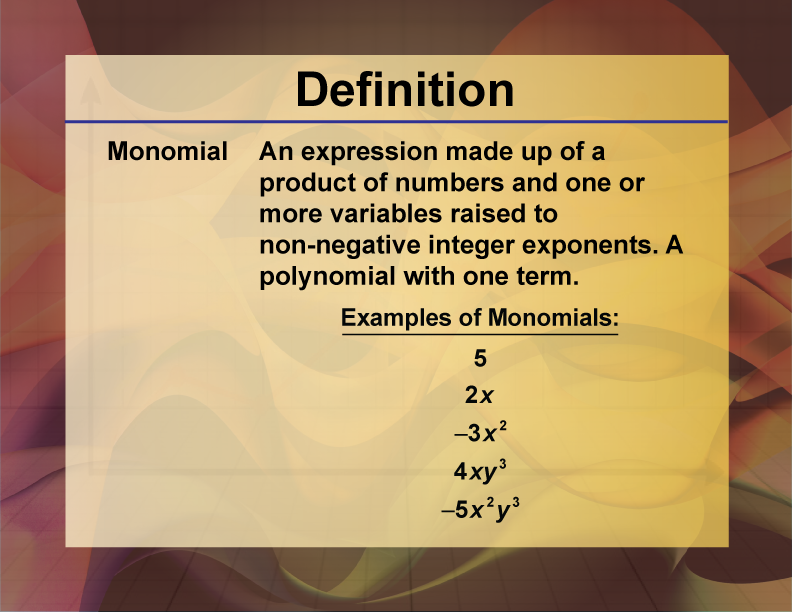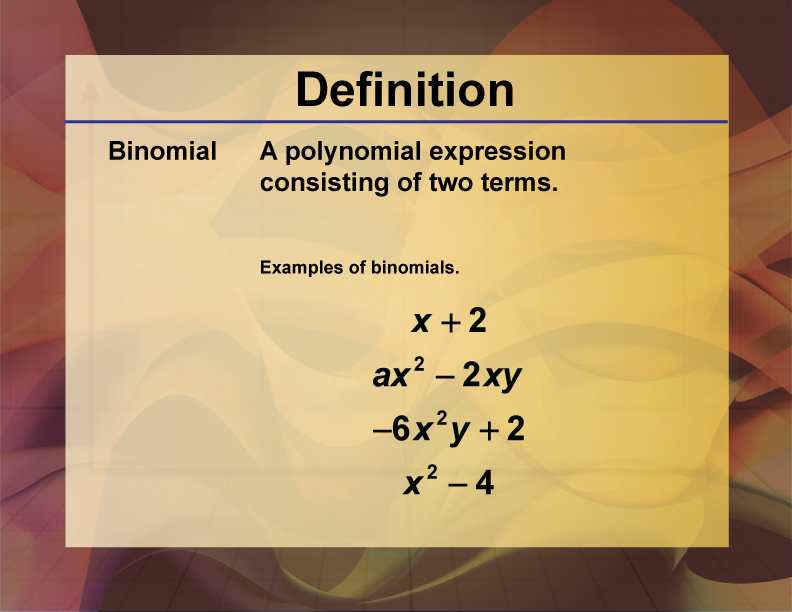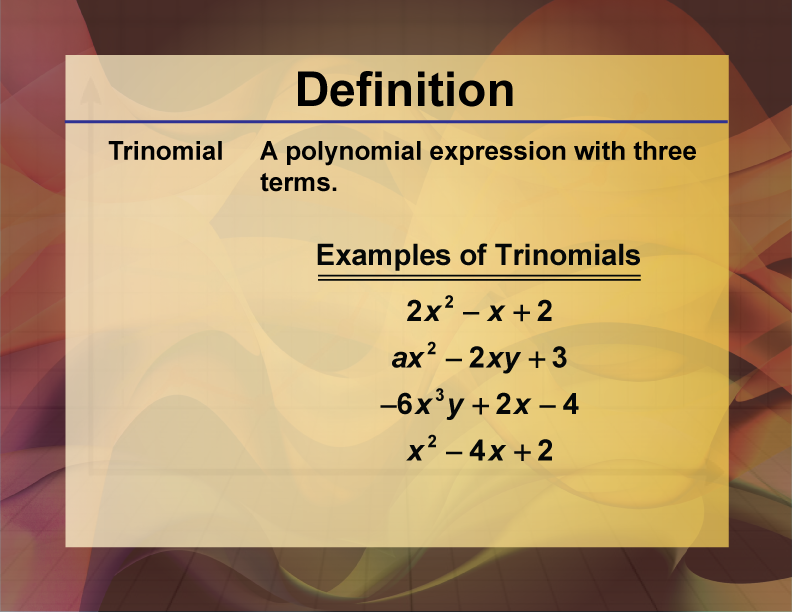# Video Tutorial--Polynomial Concepts--Video 10--Polynomials with Complex Solutions

## What Are Polynomials?

### Polynomials Are Made Up of Monomials

A monomial is a single expression that is usually the product of a number and one or more variables raised to a positive exponent power. Read the following definition.A monomial is an example of a polynomial.

### Binomials Are Made Up of Two Monomials

If you combine two monomials, you have a binomial, so long as the two monomials cannot be further added or subtracted. Read the following definition.Notice how each binomial is made up of two monomials. Here’s an example of two monomials that, when combined, result in a monomial, not a binomial.### Trinomials Are Made Up of Three Monomials

If you combine three monomials, you have a trinomial, so long as the monomials cannot be further added or subtracted. Read the following definition.Notice how each binomial is made up of three monomials.

### The Degree of a Polynomial

The term with the highest exponent determines the degree of the polynomial.

This is a polynomial of degree 1:This is a polynomial of degree 2:This is a polynomial of degree 3:This is part of a collection of video tutorials the topic of Polynomails.

## Video Transcripts

### This video has a transcript available. To see the complete collection of video transcripts, click on this link.

Common Core Standards CCSS.MATH.CONTENT.HSN.CN.C.8 5.5 minutes 9 - 12 Algebra     • Polynomials         • Polynomial Expressions         • Polynomial Functions and Equations 2021 polynomials, polynomial functions, polynomial equations, Complex Number, Complex Conjugate